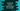# How to remove only the fractional part of a number in JavaScript## JavaScript program to remove fractional part of a number :

JavaScript provides one inbuilt method to remove the fractional part of a number. If you have one floating-point number like 1.24 and you need only the integer part i.e. 1, you can use this method. In this tutorial, I will show you how to do that in JavaScript with a simple function. In the example, I will show you how to do that with different inputs.

## JavaScript Math.trunc :

JavaScript provides one method called Math.trunc() that takes one number as its parameter and returns the integer part of that number. No matter the number is positive or negative, it cuts off the dot and everything to the right of it.

If you pass any different type of argument to it, it will implicitly convert it to a number.

## Example of Math.truc() :

``````console.log(Math.trunc(10.123));
console.log(Math.trunc(-11.123));
console.log(Math.trunc('12.123'));
console.log(Math.trunc('13.123xyz'));
console.log(Math.trunc('-14.123'));
console.log(Math.trunc(NaN));
console.log(Math.trunc(undefined));
console.log(Math.trunc('xyzabc'));
console.log(Math.trunc(15));
console.log(Math.trunc(-0));``````

### Output :

It will print the below output :

``````10
-11
12
NaN
-14
NaN
NaN
NaN
15
-0``````